PHY294H - Lecture 3

Recommended work related to Lecture 3:
Do problems: 23.9, 23.10, 23.16, 23.17

We want to undertand and control electric fields. First we need to know how to make a uniform electric field.

Uniformly charged sheet

Consider a uniformly charged sheet, with charge density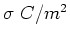. To find the electric field near such a sheet, we can use the result we found for a thin ring of charge, namely,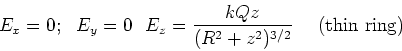(1)

The electric field for an infinite sheet is found by integrating this expression from R=0 to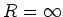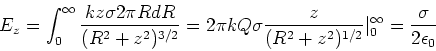(2)

Thus a uniformly charged sheet produces a uniform electric field. If two plates of equal and opposite uniform charge are placed parallel to each other, the electric field is simply a sum of the electric fields due to the individual sheets (superposition holds). This demonstrates that two parallel uniformly charged sheets (of opposite charge) have electric field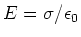between them and zero electric field outside. This is the standard method for generating a uniform electric field for use in cathode ray tubes, accelerators etc.

Charge moving in a uniform field

If a charge moves through a region of space where the electric field is uniform, it undergoes an acceleration given by,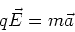(3)

Example

Consider a charge q moves with initial speed v in the x-direction. This charge enters a region of uniform electric field,, which is directed along the positive y direction. If the charge experiences this electric field for d=1cm, find the velocity of the charge after it has left the region of space in which the electric field exists.

Solution The velocity in the x-direction is unaltered, so vx=v. The velocity in the y direction is given by,

 vy = vy(0) + ay t (4)

The amount of time the charge is in the field is t=d/vx=d/v. The acceleration in the y-direction is ay = q |E|/m and vy(0) = 0. We thus have,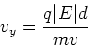(5)

Dipole in a uniform electric field

Torque

A dipole in a uniform field experiences a torque. To calculate the torque, we start with the definition of torque,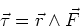(6)

The point of rotation is the center of the dipole and the force is due to the uniform electric field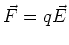where E is the electric field. In a uniform field, the force on the negative charge of the dipole is equal and opposite to the force on the positive charge of the dipole. We have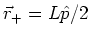,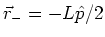and,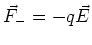. We then have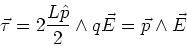(7)

Note that the magnitude of the torque is given by,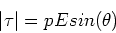(8)

where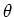is the angle between the direction of the electric field and the direction of the dipole. From this expression we see that if the dipole is parallel or antiparallel to the applied field, there is no torque. The torque is a maximum when the dipole is perpendicular to the field.

Potential energy

Using the principles of mechanics we calculate the energy of the dipole as a function of its orientation with respect to the applied field. Actually we shall calculate the change in potential energy required to rotate the dipole through an anglewith respect to the field. We have,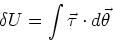(9)

Both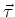and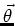are perpendicular to the plane of rotation of the dipole so we have,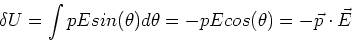(10)

The potential energy is lowest when the dipole and the electric field are aligned. The potential energy is highest when the dipole is antiparallel with the field, even though the torque is zero in that state.